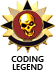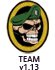Home » MODDING HQ 1.13 » v1.13 Modding, Customising, Editing » v1.13 Modding, Customising, Editing » Exact level-up salary raise formula
 Exact level-up salary raise formula Sat, 13 September 2014 20:40Charlie_MayMessages:27 Registered:March 2009
Just a small favor...

Could someone take a look at the code and give me the exact merc salary raise formula for both day, week and bi-weekly wages?

I know that merc salaries are multiplied by 1.25 at level-up (customizable in the .ini)

However, what puzzles me is how the resulting numbers are rounded up or down.

Let's say a merc gains 900\$ a day. Multiply by 1.25 and you get 1125. However, it doesn't always work like that in the game. The actual salary might be rounded up to 1150 or down to 1100. In some cases, such as the bi-weekly salaries, the numbers are rounded up or down to the thousands (11500 might become 11000 or 12000, I don't remember). Anyway, my numbers are totally arbitrary as I only did a few quick tests a while ago... I realized it would be quicker just to ask for the exact formula as it is in the code instead of figuring it out by trial and error. I need this for a little mod project.

Thanks!

EDIT : Thanks for the very thorough answer Flugente!

[Updated on: Mon, 15 September 2014 05:08] by Moderator

 Re: Exact level-up salary raise formula Sun, 14 September 2014 17:40FlugenteMessages:3456 Registered:April 2009 Location: Germany
When calculating salary, you first have to note that there is a one-day, one week and two week salary and a medical deposit, which is also kind of a salary. There is also a fifth salary type which is irrelevant here but works the same way.

New salary is old salary times 1 + (MERC_LEVEL_UP_SALARY_INCREASE_PERCENTAGE / 100). After that we round, here's the rounding function:
```UINT32 RoundOffSalary(UINT32 uiSalary)
{
UINT32 uiMultiple;

// determine what multiple value the salary should be rounded off to
if      (uiSalary <=   250) uiMultiple =    5;
else if (uiSalary <=   500) uiMultiple =   10;
else if (uiSalary <=  1000) uiMultiple =   25;
else if (uiSalary <=  2000) uiMultiple =   50;
else if (uiSalary <=  5000) uiMultiple =  100;
else if (uiSalary <= 10000) uiMultiple =  500;
else if (uiSalary <= 25000) uiMultiple = 1000;
else if (uiSalary <= 50000) uiMultiple = 2000;
else			uiMultiple = 5000;

// if the salary doesn't divide evenly by the multiple
if (uiSalary % uiMultiple)
{
// then we have to make it so, as Picard would say <- We have to wonder how much Alex gets out
// and while we're at it, we round up to next higher multiple if halfway
uiSalary = ((uiSalary + (uiMultiple / 2)) / uiMultiple) * uiMultiple;
}

return(uiSalary);
}
```

The end result has to be in [5, limit]. For daily and medical deposit the limit is 30k, for weekly an bi-weekly this is 500K.Previous Topic: 2014 Stable 1.13 Release (7435) and enemy gun choices Next Topic: Difficulty Settings in new location!
Goto Forum:

Current Time: Fri Mar 22 16:43:08 EET 2019

Total time taken to generate the page: 0.00980 seconds